Type
Quiz
Book Title
Fundamentals of Corporate Finance Standard Edition 9th Edition
ISBN 13
978-0073382395

### 978-0073382395 Chapter 8 Questions and Problems 23-28

April 3, 2019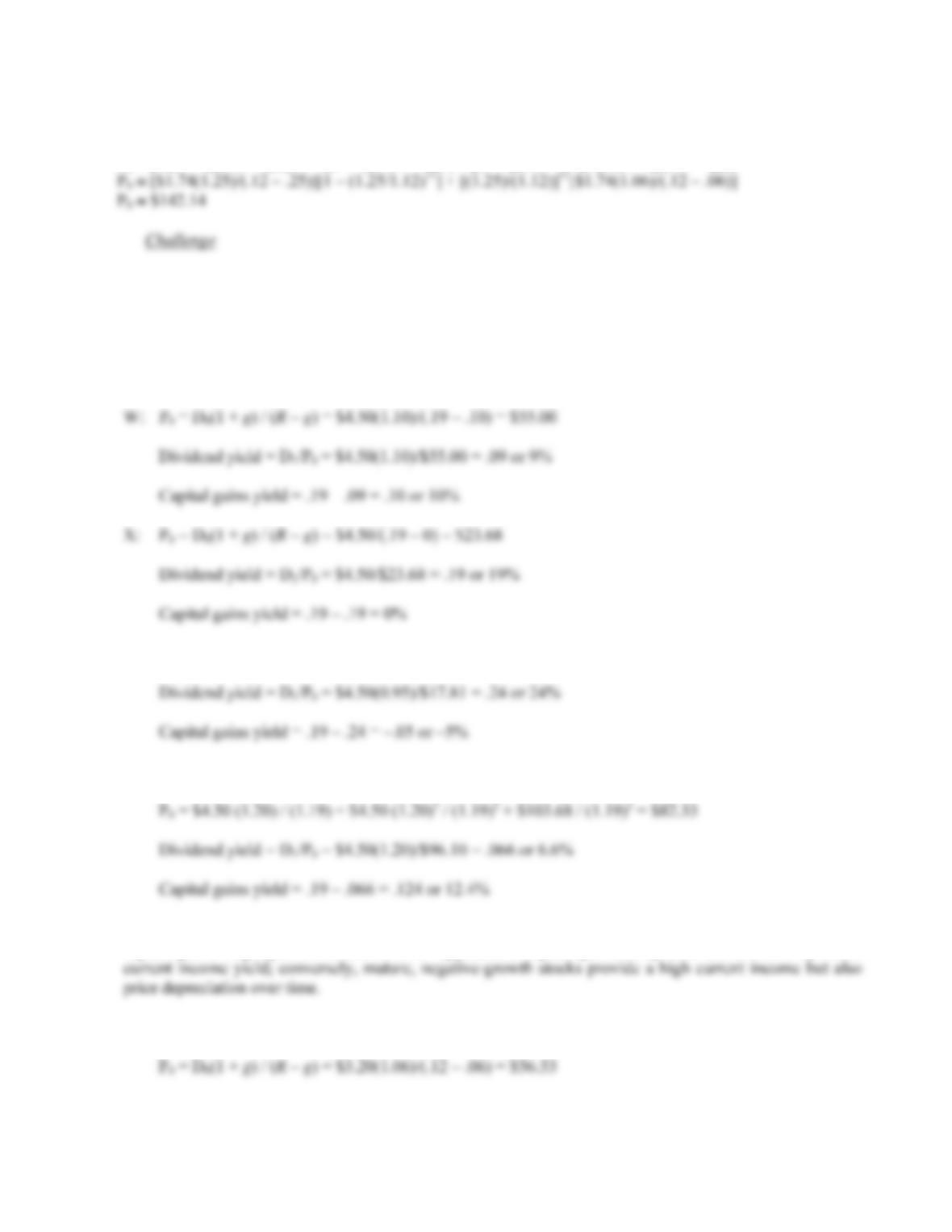B-148 SOLUTIONS
21. We can use the two-stage dividend growth model for this problem, which is:
P
0 = [D0(1 + g1)/(R – g1)]{1 – [(1 + g1)/(1 + R)]T}+ [(1 + g1)/(1 + R)]T[D0(1 + g2)/(R – g2)]
22. We are asked to find the dividend yield and capital gains yield for each of the stocks. All of the stocks have
a 15 percent required return, which is the sum of the dividend yield and the capital gains yield. To find the
components of the total return, we need to find the stock price for each stock. Using this stock price and the
dividend, we can calculate the dividend yield. The capital gains yield for the stock will be the total return
(required return) minus the dividend yield.
Y: P0 = D0(1 + g) / (Rg) = \$4.50(1 – .05)/(.19 + .05) = \$17.81
Z: P2 = D2(1 + g) / (Rg) = D0(1 + g1)2(1 + g2)/(Rg2) = \$4.50(1.20)2(1.12)/(.19 – .12) = \$103.68
P
In all cases, the required return is 19%, but the return is distributed differently between current income and
capital gains. High growth stocks have an appreciable capital gains component but a relatively small
23. a. Using the constant growth model, the price of the stock paying annual dividends will be:
P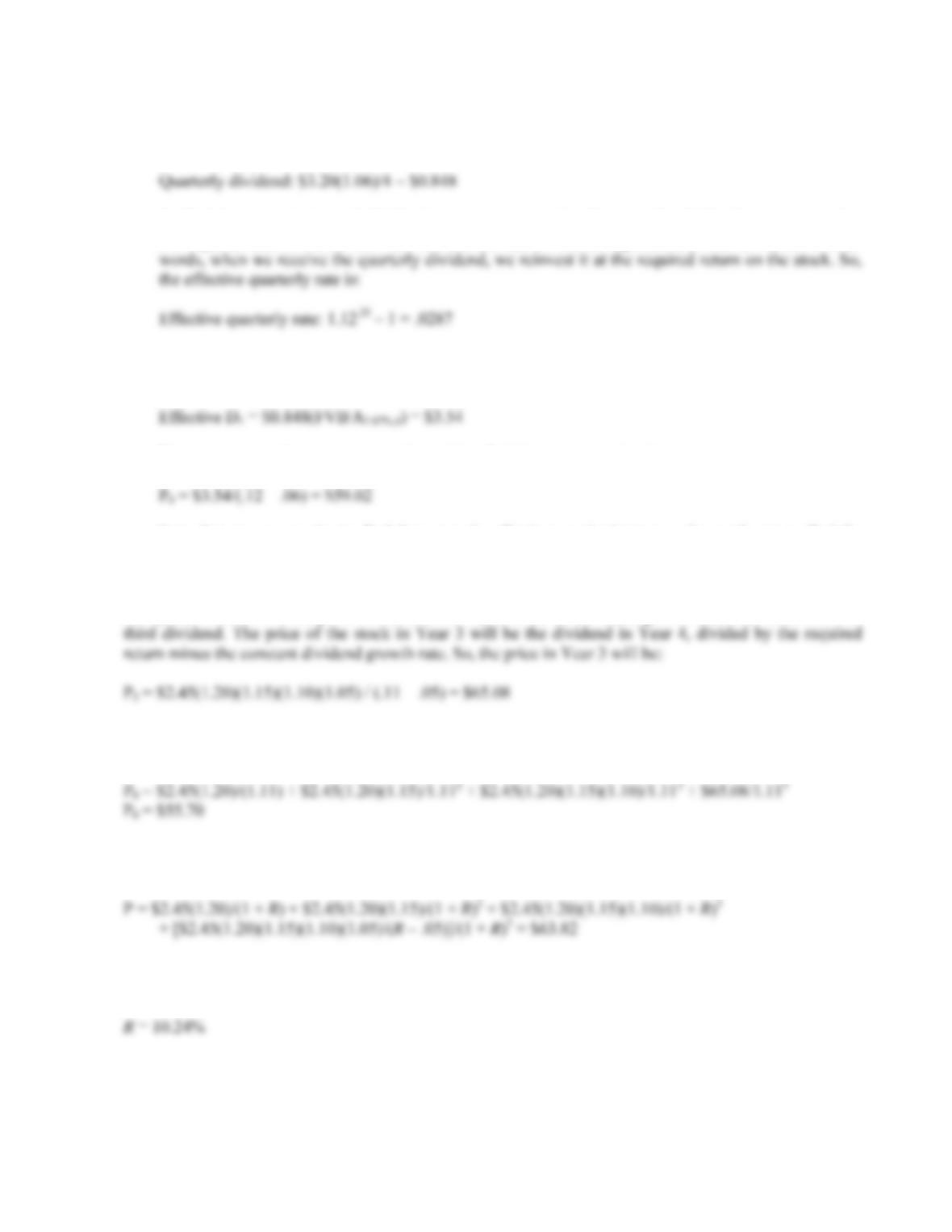CHAPTER 8 B-149
b. If the company pays quarterly dividends instead of annual dividends, the quarterly dividend will be
one-fourth of annual dividend, or:
To find the equivalent annual dividend, we must assume that the quarterly dividends are reinvested at
the required return. We can then use this interest rate to find the equivalent annual dividend. In other
The effective annual dividend will be the FVA of the quarterly dividend payments at the effective
quarterly required return. In this case, the effective annual dividend will be:
Now, we can use the constant growth model to find the current stock price as:
P
Note that we cannot simply find the quarterly effective required return and growth rate to find the
value of the stock. This would assume the dividends increased each quarter, not each year.
24. Here we have a stock with supernormal growth, but the dividend growth changes every year for the first
four years. We can find the price of the stock in Year 3 since the dividend growth rate is constant after the
The price of the stock today will be the PV of the first three dividends, plus the PV of the stock price in
Year 3, so:
P
25. Here we want to find the required return that makes the PV of the dividends equal to the current stock
price. The equation for the stock price is:
We need to find the roots of this equation. Using spreadsheet, trial and error, or a calculator with a root
solving function, we find that: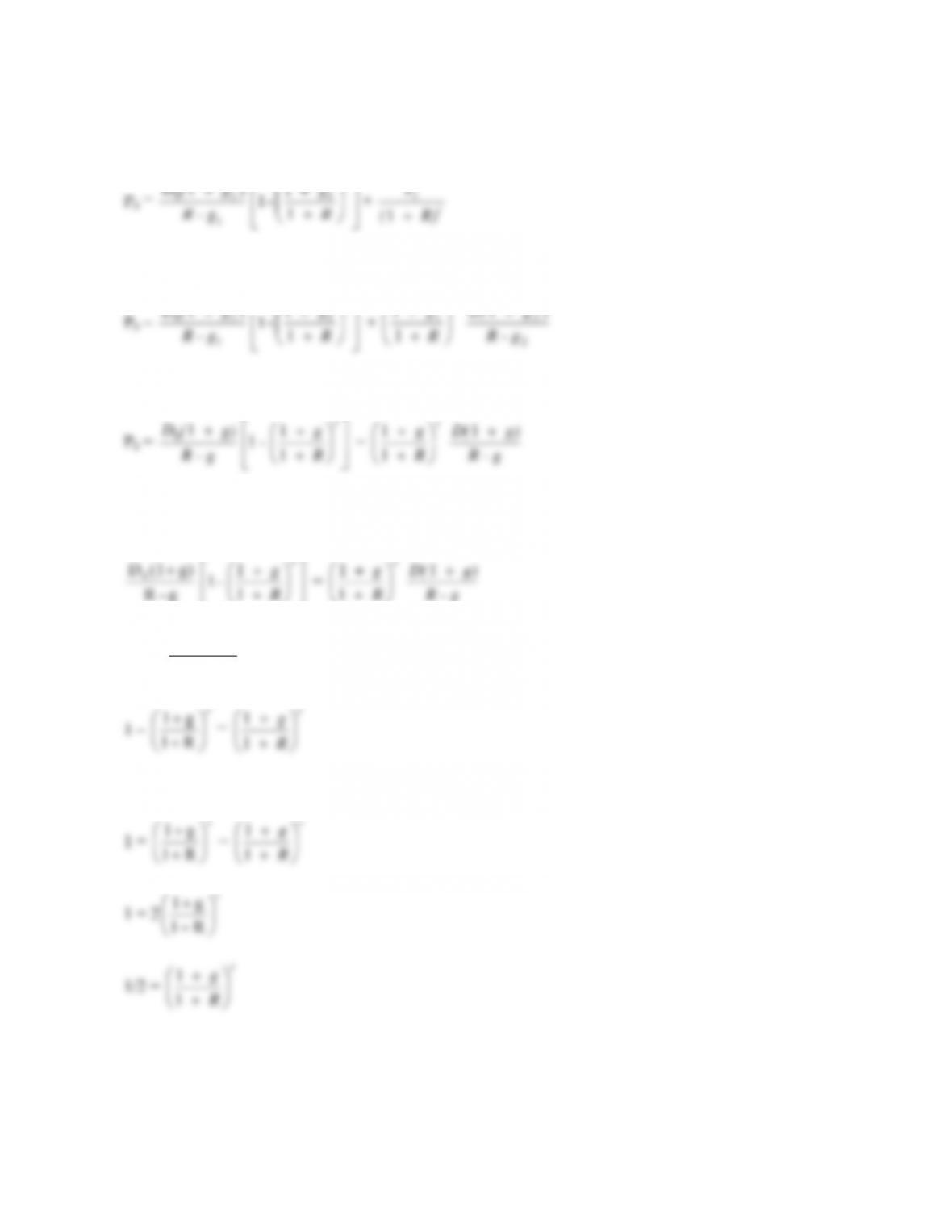B-150 SOLUTIONS
26. Even though the question concerns a stock with a constant growth rate, we need to begin with the equation
for two-stage growth given in the chapter, which is:
g
1
1
- 1 1+ t
t
R) (
P
1
We can expand the equation (see Problem 27 for more detail) to the following:
P
t
R
g
1
1
- 1 1 +
t
R
g
1
11
2
2
1
R - g
) g D(
Since the growth rate is constant, g1 = g2 , so:
P
0 = R - g
g
- 1
1
1 +
t
R
g
1
1 R - g
g) D(
1
Since we want the first t dividends to constitute one-half of the stock price, we can set the two terms on the
right hand side of the equation equal to each other, which gives us:
g - R
g) (1D0
t
R
g
1
1 R - g
g) D(
1
Since g - R
g) (1D0appears on both sides of the equation, we can eliminate this, which leaves:
1
t
R 1
1
1
Solving this equation, we get:
1 =
t
R 1
g
1
1
1 = 2
t
g
1
1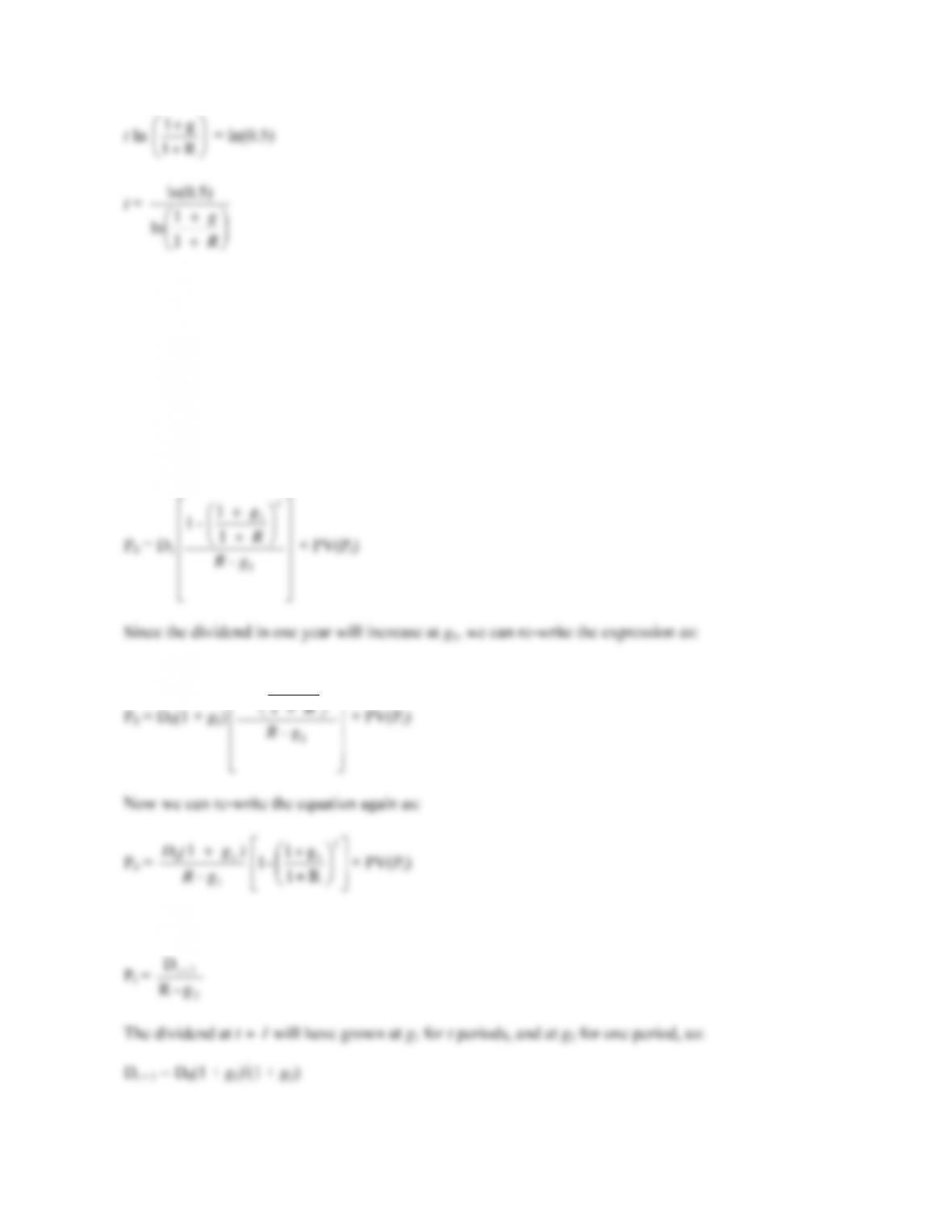CHAPTER 8 B-151
t ln
R 1
g
1
1
ln
ln(0.5)
This expression will tell you the number of dividends that constitute one-half of the current stock price.
27. To find the value of the stock with two-stage dividend growth, consider that the present value of the first t
dividends is the present value of a growing annuity. Additionally, to find the price of the stock, we need to
add the present value of the stock price at time t. So, the stock price today is:
P
0 = PV of t dividends + PV(Pt)
Using g1 to represent the first growth rate and substituting the equation for the present value of a growing
annuity, we get:
P
0 = D1
P
t
1
R 1
g 1
- 1+ PV(P
t)
To find the price of the stock at time t, we can use the constant dividend growth model, or:
P
t =
2
1 t
g - R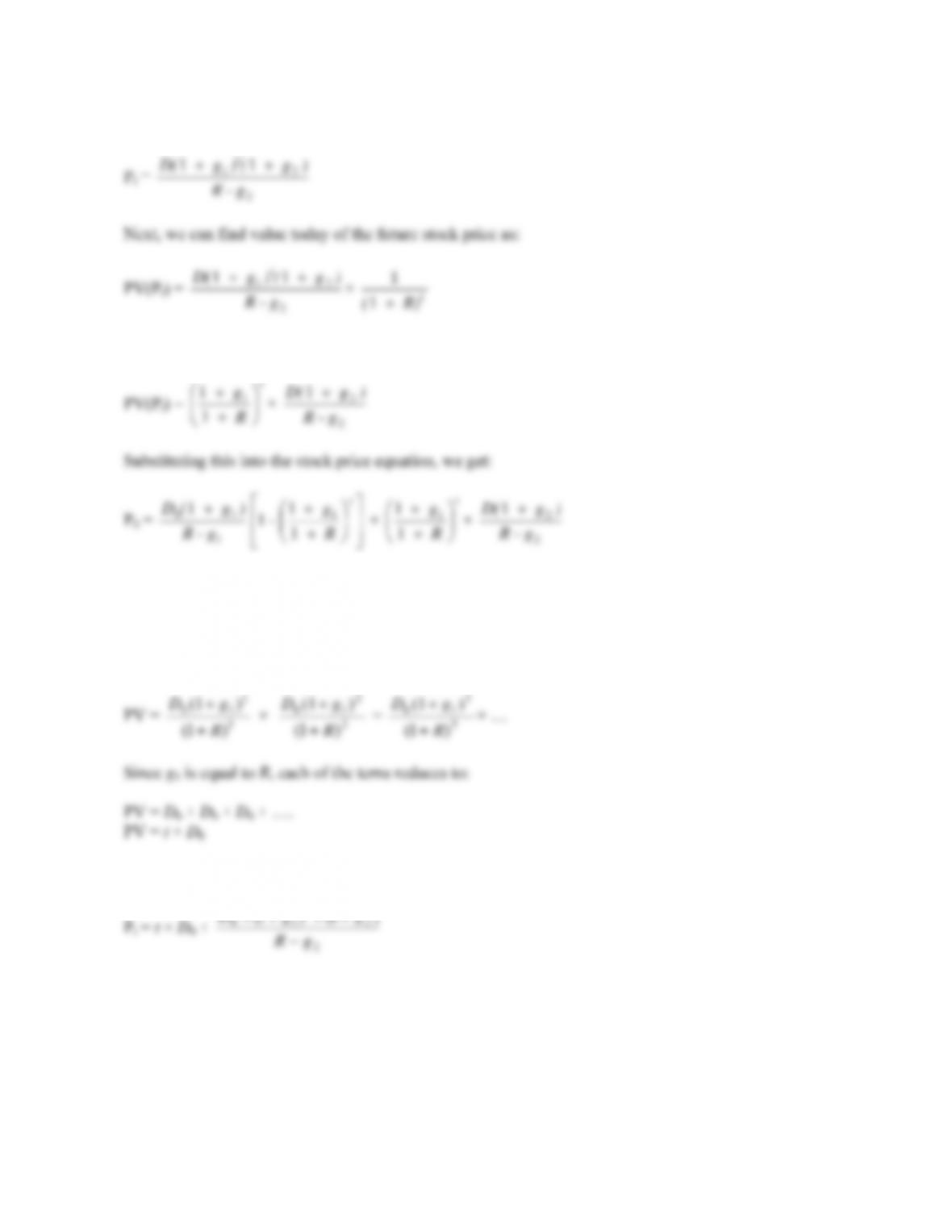B-152 SOLUTIONS
So, we can re-write the equation as:
P
t =
2
21 11
R - g
) g () g D( t× t
R) ( 1
1
which can be written as:
PV(Pt) =
t
R
g
1
g
- 1
1
11 +
t
R
g
1
11×
2
2
1
R - g
) g D(
In this equation, the first term on the right hand side is the present value of the first t dividends, and the
second term is the present value of the stock price when constant dividend growth forever begins.
28. To find the expression when the growth rate for the first stage is exactly equal to the required return,
consider we can find the present value of the dividends in the first stage as:
PV = 1
1
10
)1(
) (1
R
gD
So, the expression for the price of a stock when the first growth rate is exactly equal to the required return
is:
P
ggD t